# Power balance (Steady State AC Magnetic application)

## Introduction

In Steady state AC magnetic application, the computed physical quantities vary sinusoidally with time (at a given frequency). They are expressed by means of complex images.

The power balance allows the access to the powers dissipated by the system.

## Sub-systems definition: reminder

The system comprises one or more sub-systems like the one presented in the figure below.## Computation / results

The values calculated for each of the sub-systems and for the period T=1/f:

• the dissipated powers (mean values over a T period)
Attention: In the presence of symmetries and/or periodicities, the results correspond to the whole device, contrary to what is usually carried out in Flux.
Remember: The results usually correspond to the part of the device represented in the finite elements domain, with the exception of the magnetic flux in the coils, the forces/torques applied on mobile mechanical sets.

## Dissipated powers

For the dissipated powers, a summary of the computed quantities is presented in the table below.

Sub-system

Losses (instantaneous) (in W)

Internal
• Losses by the Joule effect in the solid conductors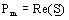, with S defined by: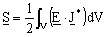• Losses by the Joule effect in the stranded conductors: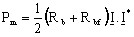(with I peak)

Electrical

Losses by the Joule effect (resistive components):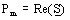, with S defined by: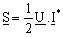(with U and I peak)